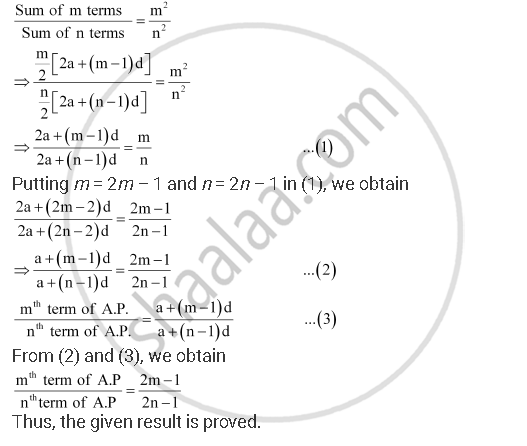Share

# The Ratio of the Sums of M and N Terms of an A.P. is M2: N2. Show that the Ratio of Mth and Nth Term is (2m – 1): (2n – 1) - Mathematics

#### Question

The ratio of the sums of m and n terms of an A.P. is m2n2. Show that the ratio of mth and nthterm is (2m – 1): (2n – 1)

#### Solution

Let a and b be the first term and the common difference of the A.P. respectively.

According to the given condition,Is there an error in this question or solution?